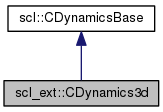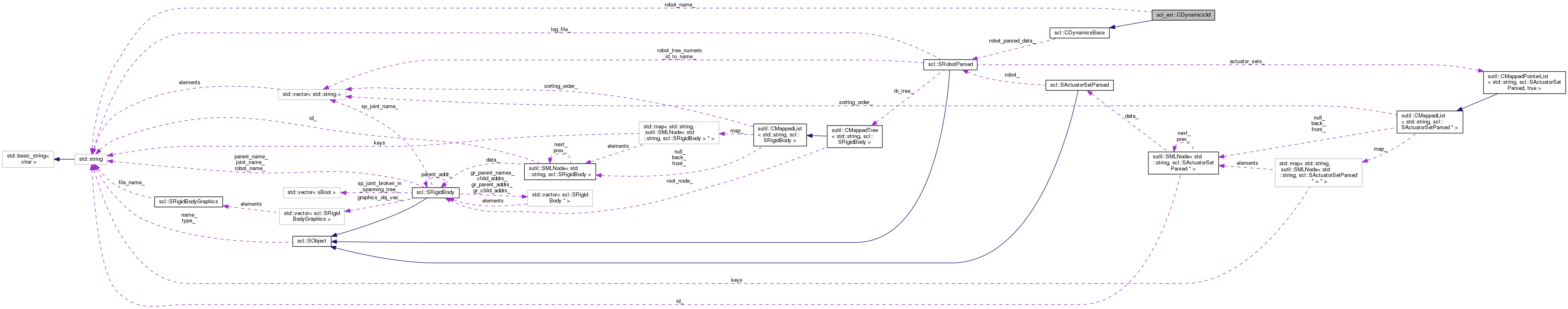SCL  1.0 Standard Control Library : Control, dynamics, physics, and simulation
scl_ext::CDynamics3d Class Reference

#include <CDynamics3d.hpp>

Inheritance diagram for scl_ext::CDynamics3d:[legend]
Collaboration diagram for scl_ext::CDynamics3d:[legend]

Public Member Functions

virtual scl::sBool computeExternalContacts (sutil::CMappedList< std::string, scl::SForce > &arg_contacts)

virtual scl::sBool integrate (scl::SRobotIO &arg_inputs, const scl::sFloat arg_time_interval)

virtual scl::sFloat computeEnergyKinetic (sutil::CMappedTree< std::string, scl::SRigidBodyDyn > &arg_tree, const Eigen::VectorXd &arg_q, const Eigen::VectorXd &arg_dq)

virtual scl::sFloat computeEnergyPotential (sutil::CMappedTree< std::string, scl::SRigidBodyDyn > &arg_tree, const Eigen::VectorXd &arg_q)

Eigen::Vector3d computeForce (std::string name)

Eigen::Vector3d computeTorque (std::string name, Eigen::Vector3d pos)

scl::sBool hasContacted (std::string name)

scl::sInt getNumContacts (std::string name)

virtual bool computeGCModel (scl::SRobotSensors const *arg_sensor_data, scl::SGcModel *arg_gc_model)

CDynamics3d ()

virtual ~CDynamics3d ()

virtual bool init (const scl::SRobotParsed &arg_robot_data)

virtual sBool computeTransformsForAllLinks (sutil::CMappedTree< std::string, SRigidBodyDyn > &arg_tree, const Eigen::VectorXd &arg_q)

virtual sBool computeTransform (SRigidBodyDyn &arg_link, const Eigen::VectorXd &arg_q)

virtual sBool computeTransformToAncestor (Eigen::Affine3d &arg_T, SRigidBodyDyn &arg_link, const SRigidBodyDyn *arg_ancestor, const Eigen::VectorXd &arg_q)

virtual sBool computeJacobianWithTransforms (Eigen::MatrixXd &arg_J, SRigidBodyDyn &arg_link, const Eigen::VectorXd &arg_q, const Eigen::Vector3d &arg_pos_local)

virtual sBool computeJacobian (Eigen::MatrixXd &arg_J, const SRigidBodyDyn &arg_link, const Eigen::VectorXd &arg_q, const Eigen::Vector3d &arg_pos_local) const

virtual sBool computeJacobian (Eigen::MatrixXd &arg_J, const SRigidBodyDyn &arg_link, const SRigidBodyDyn *arg_ancestor, const Eigen::VectorXd &arg_q, const Eigen::Vector3d &arg_pos_local)

virtual sBool computeJacobianTrans (Eigen::MatrixXd &arg_J, const SRigidBodyDyn &arg_link, const SRigidBodyDyn *arg_ancestor, const Eigen::VectorXd &arg_q, const Eigen::Vector3d &arg_pos_local)

virtual sBool computeJacobianRot (Eigen::MatrixXd &arg_J, const SRigidBodyDyn &arg_link, const SRigidBodyDyn *arg_ancestor, const Eigen::VectorXd &arg_q, const Eigen::Vector3d &arg_pos_local)

virtual sBool hasBeenInit ()

Public Attributes

cDynamicBase * c_base

Protected Attributes

sBool has_been_init_

const SRobotParsed * robot_parsed_data_

Private Attributes

std::string robot_name_

size_t ndof_

Eigen::Vector3d gravity_

Detailed Description

Computes the dynamics of a robot model using the Dynamics3d dynamics engine.

This is presently under development. Contact the author(s) for more details.

Constructor & Destructor Documentation

 scl_ext::CDynamics3d::CDynamics3d ( )

Default constructor sets the initialization state to false

 scl_ext::CDynamics3d::~CDynamics3d ( )
virtual

Default destructor does nothing

Member Function Documentation

 sFloat scl_ext::CDynamics3d::computeEnergyKinetic ( sutil::CMappedTree< std::string, scl::SRigidBodyDyn > & arg_tree, const Eigen::VectorXd & arg_q, const Eigen::VectorXd & arg_dq )
virtual

Gets the robot's kinetic energy

Parameters
 arg_tree The tree for which the transformation matrices are to be updated arg_q The current generalized coordinates. arg_dq The current generalized velocities.

Reimplemented from scl::CDynamicsBase.

 sFloat scl_ext::CDynamics3d::computeEnergyPotential ( sutil::CMappedTree< std::string, scl::SRigidBodyDyn > & arg_tree, const Eigen::VectorXd & arg_q )
virtual

Gets the robot's potential energy

Parameters
 arg_tree The tree for which the transformation matrices are to be updated arg_q The current generalized coordinates.

Reimplemented from scl::CDynamicsBase.

 virtual scl::sBool scl_ext::CDynamics3d::computeExternalContacts ( sutil::CMappedList< std::string, scl::SForce > & arg_contacts )
inlinevirtual

Calculates the collision forces for the robot to which this dynamics object is assigned.

Uses std::string based force lookup. The dynamics implementation should support this (maintain a map or something).

NOTE : The dynamics engine may delete forces from the passed mapped list at will. Do not store pointers to them at random places in your code.

NOTE TODO : Fix this:

Parameters
 arg_contacts This is where the simulator will store the contact forces. It may use the std::string to identify when to remove or re-add forces.

Reimplemented from scl::CDynamicsBase.

 virtual bool scl_ext::CDynamics3d::computeGCModel ( scl::SRobotSensors const * arg_sensor_data, scl::SGcModel * arg_gc_model )
inlinevirtual

We don't presently support this with dynamics3d

Implements scl::CDynamicsBase.

 virtual sBool scl::CDynamicsBase::computeJacobian ( Eigen::MatrixXd & arg_J, const SRigidBodyDyn & arg_link, const Eigen::VectorXd & arg_q, const Eigen::Vector3d & arg_pos_local ) const
inlinevirtualinherited

Calculates the Jacobian for the robot to which this dynamics object is assigned. dx_global_origin = Jx . dq The Jacobian is specified by a link and an offset (in task space dimensions)from that link. ** CONST VERSION : NOTE : The regular version should call this. **

Parameters
 arg_J The Jacobain will be saved here. arg_link The link at which the Jacobian is to be calculated arg_q The current generalized coordinates. arg_pos_local The offset from the link's frame (in link coordinates).

Reimplemented in scl::CDynamicsScl.

 virtual sBool scl::CDynamicsBase::computeJacobian ( Eigen::MatrixXd & arg_J, const SRigidBodyDyn & arg_link, const SRigidBodyDyn * arg_ancestor, const Eigen::VectorXd & arg_q, const Eigen::Vector3d & arg_pos_local )
inlinevirtualinherited

Calculates the Jacobian for the robot to which this dynamics object is assigned. dx_ancestor_coords = Jx . dq The Jacobian is specified by a link and an offset (in task space dimensions)from that link.

Parameters
 arg_J The Jacobain will be saved here. arg_link The link at which the Jacobian is to be calculated arg_ancestor The link up to which the Jacobian is to be calculated Pass NULL to compute the Jacobian up to the global root. arg_q The current generalized coordinates. arg_pos_local The offset from the link's frame (in link coordinates).
 virtual sBool scl::CDynamicsBase::computeJacobianRot ( Eigen::MatrixXd & arg_J, const SRigidBodyDyn & arg_link, const SRigidBodyDyn * arg_ancestor, const Eigen::VectorXd & arg_q, const Eigen::Vector3d & arg_pos_local )
inlinevirtualinherited

Calculates the Jacobian for the robot to which this dynamics object is assigned. ONLY for rotation. Ie. size(Jx) = (3,ndof) dx_ancestor_coords = Jx . dq

Parameters
 arg_J The Jacobain will be saved here. arg_link The link at which the Jacobian is to be calculated arg_ancestor The link up to which the Jacobian is to be calculated Pass NULL to compute the Jacobian up to the global root. arg_q The current generalized coordinates. arg_pos_local The offset from the link's frame (in link coordinates).
 virtual sBool scl::CDynamicsBase::computeJacobianTrans ( Eigen::MatrixXd & arg_J, const SRigidBodyDyn & arg_link, const SRigidBodyDyn * arg_ancestor, const Eigen::VectorXd & arg_q, const Eigen::Vector3d & arg_pos_local )
inlinevirtualinherited

Calculates the Jacobian for the robot to which this dynamics object is assigned. ONLY for translation. Ie. size(Jx) = (3,ndof) dx_ancestor_coords = Jx . dq

Parameters
 arg_J The Jacobain will be saved here. arg_link The link at which the Jacobian is to be calculated arg_ancestor The link up to which the Jacobian is to be calculated Pass NULL to compute the Jacobian up to the global root. arg_q The current generalized coordinates. arg_pos_local The offset from the link's frame (in link coordinates).
 virtual sBool scl::CDynamicsBase::computeJacobianWithTransforms ( Eigen::MatrixXd & arg_J, SRigidBodyDyn & arg_link, const Eigen::VectorXd & arg_q, const Eigen::Vector3d & arg_pos_local )
inlinevirtualinherited

Calculates the Jacobian for the robot to which this dynamics object is assigned. dx_global_origin = Jx . dq The Jacobian is specified by a link and an offset (in task space dimensions)from that link.

Parameters
 arg_J The Jacobain will be saved here. arg_link The link at which the Jacobian is to be calculated. Transforms are updated in this link and ancestors in the tree. arg_q The current generalized coordinates. arg_pos_local The offset from the link's frame (in link coordinates).

Reimplemented in scl::CDynamicsScl.

 virtual sBool scl::CDynamicsBase::computeTransform ( SRigidBodyDyn & arg_link, const Eigen::VectorXd & arg_q )
inlinevirtualinherited

Calculates the Transformation Matrix for the robot to which this dynamics object is assigned. x_parent_link_coords = arg_link.T_lnk_ * x_link_coords Note that the matrix is stored in the passed link data struct.

Parameters
 arg_link The link at which the transformation matrix is to be calculated arg_q The current generalized coordinates.

Reimplemented in scl::CDynamicsScl.

 virtual sBool scl::CDynamicsBase::computeTransformsForAllLinks ( sutil::CMappedTree< std::string, SRigidBodyDyn > & arg_tree, const Eigen::VectorXd & arg_q )
inlinevirtualinherited

Parameters
 arg_tree The tree for which the transformation matrices are to be updated arg_q The current generalized coordinates.

Reimplemented in scl::CDynamicsScl.

 virtual sBool scl::CDynamicsBase::computeTransformToAncestor ( Eigen::Affine3d & arg_T, SRigidBodyDyn & arg_link, const SRigidBodyDyn * arg_ancestor, const Eigen::VectorXd & arg_q )
inlinevirtualinherited

Calculates the Transformation Matrix for the robot to which this dynamics object is assigned. x_ancestor_link_coords = arg_link.T_lnk_ * x_link_coords

Note that the local transformation matrices are updated in all the link data structs from arg_link to arg_ancestor.

Also note that there are two origins, robot origin, which actually has a node allocated for it. And the global scl origin, for which you should pass in a NULL as ancestor.

Parameters
 arg_T The transformation matrix in which to store the answer arg_link The link at which the transformation matrix is to be calculated arg_ancestor The link up to which the transformation matrix is to be calculated Pass NULL to compute the transform up to the global root. arg_q The current generalized coordinates.

Reimplemented in scl::CDynamicsScl.

virtual

NOTE TODO : This is obsolete. WILL BE DELETED SOON!

Gives an id for a link name.

Enables using calculateJacobain without:

1. Storing any dynamic-engine specific objects in the controller.
2. Using inefficient repeated string based lookup (usually with maps)
 virtual sBool scl::CDynamicsBase::hasBeenInit ( )
inlinevirtualinherited

Initialization state

 bool scl_ext::CDynamics3d::init ( const scl::SRobotParsed & arg_robot_data )
virtual

Initializes the dynamics to be computed for a specific robot.

Returns, true : Succeeds in creating a dynamics object

false : If it can't find the robot or if the information is inconsistent with what the implementation requires, it returns false

Implements scl::CDynamicsBase.

 sBool scl_ext::CDynamics3d::integrate ( scl::SRobotIO & arg_inputs, const scl::sFloat arg_time_interval )
virtual

Integrates the robot's state. Uses the given applied forces, torques, positions and velocities and its internal dynamic model to compute new positions and velocities. Operates on the SRobotIO data structure.

Parameters
 arg_inputs The existing generalized coordinates, velocities and accelerations + The generalized forces + task (euclidean) forces and the list of contact points and links. arg_time_interval The time across which the system should integrate the dynamics. Could take fixed steps or dynamic ones in between. Up to the integrator implementation.

Reimplemented from scl::CDynamicsBase.

Member Data Documentation

 Eigen::Vector3d scl_ext::CDynamics3d::gravity_
private

The gravity acting on the robot

 sBool scl::CDynamicsBase::has_been_init_
protectedinherited

True if the dynamics object has been initialized for a given robot

 size_t scl_ext::CDynamics3d::ndof_
private

The robot's degrees of freedom

 std::string scl_ext::CDynamics3d::robot_name_
private

The robot's name

 const SRobotParsed* scl::CDynamicsBase::robot_parsed_data_
protectedinherited

A read-only pointer to access parsed data

The documentation for this class was generated from the following files:
• /home/samir/Code/control/scl.git/src/scl_ext/dynamics/dynamics3d/CDynamics3d.hpp
• /home/samir/Code/control/scl.git/src/scl_ext/dynamics/dynamics3d/CDynamics3d.cpp